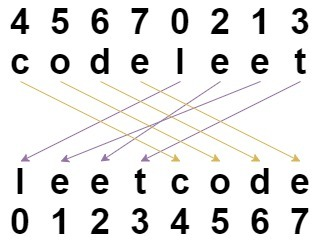# 算法题# 面试题 01.03. URL 化

URL 化。编写一种方法，将字符串中的空格全部替换为 %20。假定该字符串尾部有足够的空间存放新增字符，并且知道字符串的“真实”长度。（注：用 Java 实现的话，请使用字符数组实现，以便直接在数组上操作。）

class Solution {    public String replaceSpaces(String S, int length) {            char[] ch = new char[length * 3];        int index = 0;        for (int i = 0; i < length; i++) {            char c = S.charAt(i);            if (c == ' ') {                ch[index++] = '%';                ch[index++] = '2';                ch[index++] = '0';            } else {                ch[index] = c;                index++;            }        }        return new String(ch, 0, index);    }}

# 1528. 重新排列字符串class Solution {    public String restoreString(String s, int[] indices) {        int length = s.length();        char[] result = new char[length];        for (int i = 0; i < length; i++) {            result[indices[i]] = s.charAt(i);        }        return new String(result);    }}## 评论